# How to find the Equation of A Circle?

• Last Updated : 30 May, 2022

In geometry, a circle can be defined as the set of all points that are equidistant from a fixed point in the plane, where the fixed point is the center of the circle. The radius is defined as the distance between any point on the circumference of a circle and the center of the circle. The largest chord that passes through the center of the circle; this diameter is referred to as the circle’s diameter.

### Equation of a Circle

A circle can be defined as a closed curve traced out by a point that moves in a plane such that the distance from the given point in that plane is always constant. By using the equation of a circle, we can describe a circle in an algebraic way when the center of the circle and its radius length is known. We require an algebraic equation to represent the circle on a Cartesian plane. So, to find the position of the circle on a Cartesian plane, we require the coordinates of the center and the length of its radius.

The standard form of the equation of a circle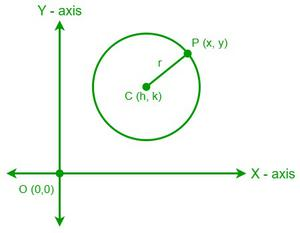Let’s consider a circle with a center (h, k) and P (x, y) to be any point on the circumference of the circle for finding the standard form of the equation. From the definition of the radius of the circle,

The radius of the given circle (r) = CP

We can find the value of CP By using the formula for the distance between two points i.e.,

CP = r = √[(x – h)2 + (y – k)2]

Now, by squaring on both sides we get,

(x – h)2 + (y – k)2 = r2

Hence, the standard form of the equation of a circle is,

(x – h)2 + (y – k)2 = r2

Where (h, k) is the center of the circle and r is the length of the radius.

• Equation of the circle with the center at the origin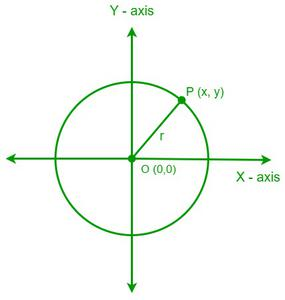Let’s consider that the center of the circle lies at the origin (0, 0) and its radius is “r”. Now, the distance between the origin and any point P (x, y) on the circle is the radius of the circle, i.e.,

OP = r

By using the formula for the distance between two points,

OP = r = √[(x – 0)2 + (y – 0)2]

Now, by squaring on both sides,

r2 = x2 + y2

Hence, the equation of the circle with the center at the origin is

x2 + y2 = r2

• Equation of the circle passing through the origin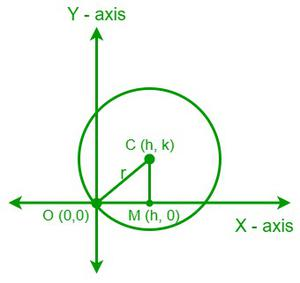Let’s consider that a circle with a center C (h, k) and a radius “r” is passing through the origin. The distance between the center of the circle and the origin is the radius of the circle, i.e.,

OC = r

Draw a line from the center to a point M (h, 0) on the X-axis such that CM is perpendicular to OM.

From the Pythagorean theorem;

OC2 = OM2 + CM2

r2 = h2 + k2

Hence, the equation for the circle passing through the origin is,

r2 = h2 + k2

• Equation of the circle with the center lying on the X-axis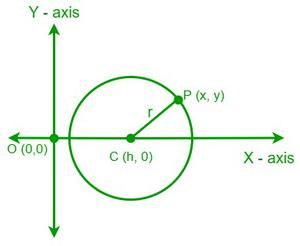Assume that the center of circle C (h, 0) is on the X-axis and its radius is “r.”

Now, the distance between the center of the circle and any point P (x, y) on the circle is the radius of the circle, i.e.,

CP = r

By using the formula for the distance between two points, we get

CP = r = √[(x – h)2 + (y – 0)2]

Now, by squaring on both sides, we get

r2 = (x – h)2 +y2

Hence, the equation for the circle passing through the origin is,

(x – h)2 +y2 = r2

• Equation of the circle with the center lying on the Y-axis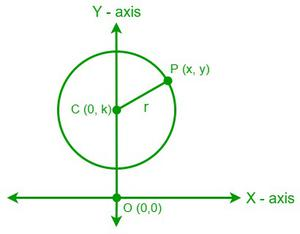Let’s consider that the center of circle C (0, k) lies on the Y-axis and its radius is “r”. Now, the distance between the center of the circle and any point P (x, y) on the circle is the radius of the circle, i.e.,

CP = r

By using the formula for the distance between two points,

CP = r = √[(x – 0)2 + (y – k)2]

Now, by squaring on both sides we get,

r2 = x2 +(y – k)2

Hence, the equation of the circle with the center lying on the Y-axis is,

x2 +(y – k)2 = r2

• Equation of the circle touching the X-axis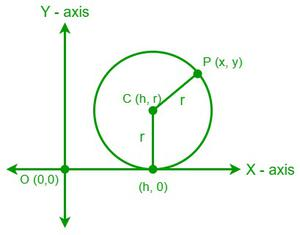Let us consider that the center of circle C (h, r) is touching the X-axis and its radius is “r”. Now, the y-coordinate of the center of the circle touching the X-axis is equal to the radius “r”.

Now, the distance between the center of the circle C (h, r) and any point P (x, y) on the circle is the radius of the circle, i.e.,

CP = r

By using the formula for the distance between two points, we get

CP = r = √[(x – h)2 + (y – r)2]

Now, by squaring on both sides we get,

r2 = (x – h)2 +(y – r)2

Hence, the equation of the circle touching the X-axis is

(x – h)2 +(y – r)2 = r2

• Equation of the circle touching the Y-axis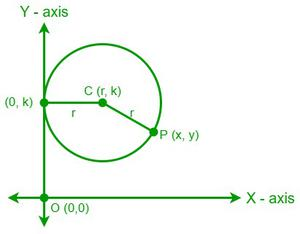Let’s consider that the center of circle C (r, k) is touching the Y-axis and its radius is “r”. Now, the x-coordinate of the center of the circle touching the Y-axis is equal to the radius “r”. Now, the distance between the center of the circle C (r, k) and any point P (x, y) on the circle is the radius of the circle, i.e.,

CP = r

By using the formula for the distance between two points,

CP = r = √[(x – r)2 + (y – k)2]

Now, by squaring on both sides we get,

r2 = (x – r)2 +(y – k)2

Hence, the equation of the circle touching the Y-axis is

(x – r)2 +(y – k)2 = r2

• Equation of the circle touching both the X-axis and the Y-axis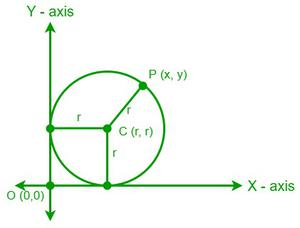Let’s assume that a circle with a center C (r, r) with a radius “r” is touching both the X-axis and the Y-axis. As the circle touches both the X-axis and the Y-axis, the x and y coordinates of the center become equal to the radius of the circle “r”.

Now, the distance between the center of the circle C (r, r) and any point P (x, y) on the circle is the radius of the circle, i.e.,

CP = r

By using the formula for the distance between two points,

CP = r = √[(x – r)2 + (y – r)2]

Now, by squaring on both sides we get

r2 = (x – r)2 +(y – r)2

Hence, the equation of the circle touching both the X-axis and Y-axis is

(x – r)2 +(y – r)2 = r2

General Equation of a Circle

The general equation of a circle is given as,

x2+ y2 + 2gx + 2fy + c = 0

For all values of g, f, and c.

x2 + y2 + 2gx + 2fy + c = 0

By adding g2 + f2 on both sides we get,

x2 + y2 + 2gx + 2fy + c + g2 + f2 = g2 + f2

Now, by arranging the equation we get

(x2 + 2gx + g2) + (y2 + 2fy + f2) = g2 + f2 – c

We know that, (x + g)2 =(x2 + 2gx + g2) and (y + f)2 = (y2 + 2fy + f2). So, by substituting these values in the above equation we get

(x + g)2 + (y + f)2 = g2 + f2 – c

By comparing the above equation with the standard form of the equation we get

h = -g, k = -f and r2 = g2 + f2 – c

Where (h, k) are the coordinates of the center of a circle and “r” is its radius.

Hence, the equation x2+ y2 + 2gx + 2fy + c = 0 represents a circle having center (-g, -f) and radius r equal to r2 = g2 + f2 – c

• The radius of a circle is real if g2 + f2 > c.
• The radius of a circle becomes imaginary if g2 + f2 < c. Hence, the circle will be having a real center and an imaginary radius.

### Sample Problems

Problem 1: Find the equation of a circle with the center at the origin and having a radius of 6 units.

Solution:

Given data,

Center of the circle = (0,0) and the radius = 6 units

We know that,

The equation of a circle whose center is at the origin is,

x2 + y2 = a2

Where a is the radius of the circle.

So, the equation of the circle having a radius of 6 units is,

x2 + y2 = (6)2

x2 + y2 = 36

Hence, the equation of the given circle is x2 + y2 = 36

Problem 2: Find the radius and the center of a circle if the equation of the circle is x2 + y2 – 8x + 6y + 9 = 0

Solution:

Given data,

The equation of the circle is x2 + y2 – 8x + 6y + 9 = 0

We can see the  given equation is in the form of  x2+ y2 + 2gx + 2fy + c = 0

Now by comparing the both equations,

2g = -8 ⇒ g = -4

2f = 6 ⇒ f = 3

c = 9

Center of the circle = (-g, -f) = (4, -3)

Radius of the circle (a) = √(g2 + f2 – c)

⇒ a = √[(-4)2 + 32 – 9] = √(16 + 9 – 9)

a = √16 = 4 units

Hence, the center of the given circle is (4, -3) and its radius is 4 units.

Problem 3: Find the equation of the circle whose center is (5, 0), and radius is 7 units.

Solution:

Given data,

Center of a circle = (5, 0)

Radius of the circle = 7 units

The general equation of a circle with center (h, k) and radius “a” units is,

(x – h)2 + (y – k)2 = a2

Now, the equation of the given circle is,

(x – 5)2 + (y – 0)2 = (7)2

⇒ x2 – 10x + 25 + y2 = 49

⇒ x2 + y2 – 10x – 24 = 0

Therefore, the equation of the given circle is x2 + y2 – 10x – 24 = 0

Problem 4: Find the equation of the circle center passing through the Y-axis whose center is (0,-6) and radius is 10 units.

Solution:

Given data,

Center of a circle = (0,-6)

The radius of the circle = 10 units.

We know that,

The equation of the circle with the center lying on the Y-axis is,

x2 +(y – k)2 = r2

x2 + (y + 6)2 = (10)2

x2 + y2 + 12y + 36 = 100

x2 + y2 + 12y – 64 = 0

Hence, the equation of the circle is x2 + y2 + 12y – 64 = 0

Problem 5: Find the equation of the circle with a radius of 6 inches that touches both the X-axis and the Y-axis.

Solution:

Given data,

The radius of the circle = 6 inches.

As the circle touches both the X-axis and the Y-axis, the x and y coordinates of the center become equal to the radius of the circle “r”.

Hence, the center of the circle = (6,6)

We have,

The equation of the circle touching both the X-axis and Y-axis is

(x – r)2 +(y – r)2 = r2

(x – 6)2 + (y – 6)2 = 62

x2 -12x + 36 + y2 – 12y + 36 = 36

x2 + y2 -12x -12y + 36 = 0

Hence, the equation of the circle touching both the X-axis and Y-axis is x2 + y2 -12x -12y + 36 = 0

Problem 6: Find the equation of the circle with the center at (-7, 4) and the radius of 4 units and touch the X-axis.

Solution:

Given data,

Center of the circle = (-7, 4)

The radius of the circle = 4 units

The equation of the circle touching the X-axis is

(x – h)2 +(y – r)2 = r2

(x + 7)2 + (y – 4)2 = 42

x2 + 14x + 49 + y2 – 8y + 16 = 16

x2 + y2 + 14x – 8y + 49 = 0

Hence, the  equation of the circle touching the X-axis is x2 + y2 + 14x – 8y + 49 = 0

Problem 7: Find the equation of the circle with a radius of 5 units and is touching the Y-axis at the point (0,-3).

Solution:

Given data,

The radius of the circle = 5 units.

The circle is touching the Y-axis at (0,-3)

Now, the x-coordinate of the center of the circle touching the Y-axis is equal to the radius “4 units”.

Hence, the coordinates of the center = (5, -7)

We have,

The equation of the circle touching the Y-axis is

(x – r)2 + (y – k)2 = r2

(x – 5)2 + (y + 3)2 = 52

x2 – 10x + 25 + y2 + 6y + 9 = 25

x2 + y2 -10x + 6y + 25 = 0

Hence, the equation of the circle touching the Y-axis is x2 + y2 -10x + 6y + 25 = 0

My Personal Notes arrow_drop_up
Recommended Articles
Page :